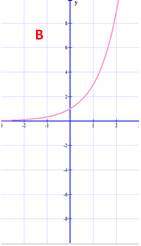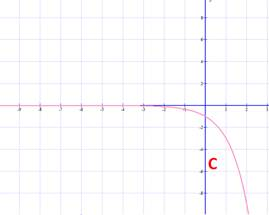### Sample Problem

Fill out the following table if f(x)=3-x (keep all non-integer answers in reduced fraction form)

 x -3 -2 -1 0 1 2 3 f(x)

Does A, B, C or D accurately represent f(x)? (type your answer, A, B, C, or D in the blank)#### Solution

F(x)=3-x, plug all the x-values in

 x -3 -2 -1 0 1 2 3 f(x) 27 9 3 1 1/3 1/9 1/27

As x gets larger, the y (or f(x)) values are getting smaller and smaller but they do not seem to

go below the x-axis (or below 0), the graph that represents the function f is A.